Test: Probability- Assertion & Reason Type Questions

# Test: Probability- Assertion & Reason Type Questions - Class 9

Test Description

## 10 Questions MCQ Test Online MCQ Tests for Class 9 - Test: Probability- Assertion & Reason Type Questions

Test: Probability- Assertion & Reason Type Questions for Class 9 2023 is part of Online MCQ Tests for Class 9 preparation. The Test: Probability- Assertion & Reason Type Questions questions and answers have been prepared according to the Class 9 exam syllabus.The Test: Probability- Assertion & Reason Type Questions MCQs are made for Class 9 2023 Exam. Find important definitions, questions, notes, meanings, examples, exercises, MCQs and online tests for Test: Probability- Assertion & Reason Type Questions below.
Solutions of Test: Probability- Assertion & Reason Type Questions questions in English are available as part of our Online MCQ Tests for Class 9 for Class 9 & Test: Probability- Assertion & Reason Type Questions solutions in Hindi for Online MCQ Tests for Class 9 course. Download more important topics, notes, lectures and mock test series for Class 9 Exam by signing up for free. Attempt Test: Probability- Assertion & Reason Type Questions | 10 questions in 20 minutes | Mock test for Class 9 preparation | Free important questions MCQ to study Online MCQ Tests for Class 9 for Class 9 Exam | Download free PDF with solutions
 1 Crore+ students have signed up on EduRev. Have you?
Test: Probability- Assertion & Reason Type Questions - Question 1

### Direction: In the following questions, a statement of Assertion is given followed by a corresponding statement of Reason just below it. Of the statements, mark the correctanswer asAssertion : Two well balanced dice are rolled and the numbers that turn up are observed. Then the number of elements in sample space is 12.Reason : When two dice are rolled, number of elements in sample space is 6 x 6.

Detailed Solution for Test: Probability- Assertion & Reason Type Questions - Question 1
When two dice are thrown simultaneously, thus number of event can be 62 = 36 because each die has 1 to 6 number on its faces. Then the possible outcomes are shown in the below table.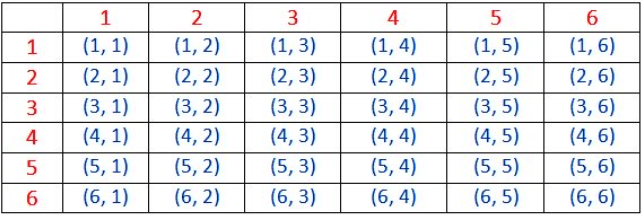Test: Probability- Assertion & Reason Type Questions - Question 2

### Direction: In the following questions, a statement of Assertion is given followed by a corresponding statement of Reason just below it. Of the statements, mark the correctanswer asAssertion : Two coins are tossed. Number of elements in the sample space is 4.Reason : When a coin is tossed n times then the number of elements in its sample space is 2n.

Detailed Solution for Test: Probability- Assertion & Reason Type Questions - Question 2
Sample space S = {(H, H), (H, T),(T, H),(T, T)}

Number of sample space n(S) = 4

D = {(T, T)}

n(D) = 1

Test: Probability- Assertion & Reason Type Questions - Question 3

### Direction: In the following questions, a statement of Assertion is given followed by a corresponding statement of Reason just below it. Of the statements, mark the correctanswer asAssertion : A fair die is rolled. Then the probability of getting an even number is 1/2 and probability of getting an odd number is 1/2.Reason : Possible outcomes when a fair die is rolled is (1, 2, 3, 4, 5, 6).

Detailed Solution for Test: Probability- Assertion & Reason Type Questions - Question 3
Possible outcomes when a die is thrown = {1, 2, 3, 4, 5, 6}

P (getting an odd number) = 3/6 = 1/2

P (getting an even number) = 3/6 = 1/2

Test: Probability- Assertion & Reason Type Questions - Question 4

Direction: In the following questions, a statement of Assertion is given followed by a corresponding statement of Reason just below it. Of the statements, mark the correct

Assertion : If E1, E2 , .........., En are n elementary events associated to a random experiment, then P(E1) + P(E2) PE +.....+ P(En) = 1

Reason : For any event ‘A’ associated to an experiment, we have -1 ≤ P(A) ≤ 1

Detailed Solution for Test: Probability- Assertion & Reason Type Questions - Question 4
Given = p(E1) + p(E2) = X

p(E3) = 1/3

In an elementary event the sum of all the events is equal to 1

p(E1) + p(E2) + p(E3) = 1

X + 1/3 = 1

X = 1 - 1/3

X = 2/3

Test: Probability- Assertion & Reason Type Questions - Question 5

Direction: In the following questions, a statement of Assertion is given followed by a corresponding statement of Reason just below it. Of the statements, mark the correct

Assertion : In a class there are x boys. and y girls, A student is selected at random, then the probability of selecting a girl is y/x.

Reason : Probability of an event E of an experiment is ratio of the number of trials in which event E has happened to the total number of trials.

Detailed Solution for Test: Probability- Assertion & Reason Type Questions - Question 5
P (selecting a girl) = y/(x+y)
Test: Probability- Assertion & Reason Type Questions - Question 6

Direction: In the following questions, a statement of Assertion is given followed by a corresponding statement of Reason just below it. Of the statements, mark the correct

Assertion : A die is thrown. Let E be the event that number appears on the upper face is less than 1, then P(E) = 1/6

Reason : Probability of impossible event is 0.

Detailed Solution for Test: Probability- Assertion & Reason Type Questions - Question 6
When a die is thrown, then number of outcomes are 1, 2, 3, 4, 5, 6

P(number appear on the upper face is less than 1) = 0

Test: Probability- Assertion & Reason Type Questions - Question 7

Direction: In the following questions, a statement of Assertion is given followed by a corresponding statement of Reason just below it. Of the statements, mark the correct

Assertion : Tossing a coin 50 times is called an event.

Reason : The possible outcomes of an experiment are called events.

Detailed Solution for Test: Probability- Assertion & Reason Type Questions - Question 7
Tossing a coin 50 times is called an experiment.
Test: Probability- Assertion & Reason Type Questions - Question 8

Direction: In the following questions, a statement of Assertion is given followed by a corresponding statement of Reason just below it. Of the statements, mark the correct

Assertion : A coin is tossed two times. Probability of getting at least two heads is 1/4.

Reason : When a coin is tossed two times, then the sample space is {HH, HT, TH, TT}

Detailed Solution for Test: Probability- Assertion & Reason Type Questions - Question 8
Number of total outcomes when a coin is tossed 2 times i.e., {HH, HT, TH, TT} = 4

P(getting at least two heads) = 1/4

Test: Probability- Assertion & Reason Type Questions - Question 9

A die is rolled if the number on a die is even then a coin is tossed once and if the number on a die is odd, then a coin is tossed twice. Match the events in Column-I with their probabilities in Column-II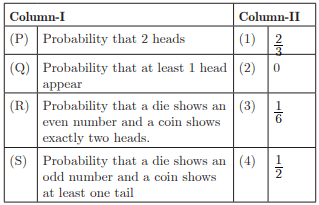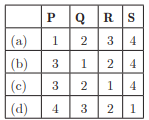Detailed Solution for Test: Probability- Assertion & Reason Type Questions - Question 9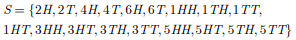(P)

n(S) = 18

Let E be the event that 2 heads appears then

n (E) = 3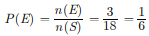(Q)

n (S) = 18

Let F be the event that at least 1 head appears

then n(F) = 12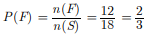(R)

n(S) = 18

Let G be the event that die shows an even number

and coin shows exactly two heads. Then n(G) = 0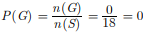(S)

n(S) = 18

Let H be the event that die shows an odd number and coin shows at least one tail.

n(H) = 9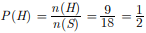Test: Probability- Assertion & Reason Type Questions - Question 10

A NGO selected 2000 families at random and surveyed them to determine number of children in a family. The data is given below: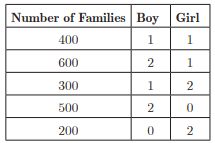If one family is chosen at random then, match the Column-I with their corresponding probabilities in Column-II.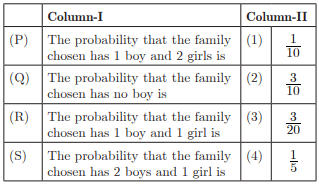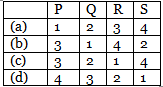Detailed Solution for Test: Probability- Assertion & Reason Type Questions - Question 10

(P) Let E be the event that chosen family has 1 boy and 2 girls

Hence, n(E) = 300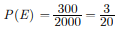(Q) Let F be the event that chosen family has no boy

n(F) = 200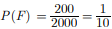(R) Let G be the event that chosen family has 1 boy and 1 girl

Hence, n(G) = 400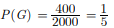(S) Let H be event that chosen family has 2 boys and 1 girl

Hence, n(H) = 600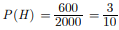## Online MCQ Tests for Class 9

399 tests
Information about Test: Probability- Assertion & Reason Type Questions Page
In this test you can find the Exam questions for Test: Probability- Assertion & Reason Type Questions solved & explained in the simplest way possible. Besides giving Questions and answers for Test: Probability- Assertion & Reason Type Questions, EduRev gives you an ample number of Online tests for practice

399 tests